Courses

# Test : Trigonometric Functions And Identities - 3

## 20 Questions MCQ Test Topic-wise Tests & Solved Examples for IIT JAM Mathematics | Test : Trigonometric Functions And Identities - 3

Description
This mock test of Test : Trigonometric Functions And Identities - 3 for Mathematics helps you for every Mathematics entrance exam. This contains 20 Multiple Choice Questions for Mathematics Test : Trigonometric Functions And Identities - 3 (mcq) to study with solutions a complete question bank. The solved questions answers in this Test : Trigonometric Functions And Identities - 3 quiz give you a good mix of easy questions and tough questions. Mathematics students definitely take this Test : Trigonometric Functions And Identities - 3 exercise for a better result in the exam. You can find other Test : Trigonometric Functions And Identities - 3 extra questions, long questions & short questions for Mathematics on EduRev as well by searching above.
QUESTION: 1

Solution:
QUESTION: 2

Solution:
QUESTION: 3

### If the equation a1 - a2, cos 2x + a3, sin2x = 1 is satisfied by every real value of x then the number of possible values of the triplet (a1,a2, a3) is

Solution:
QUESTION: 4

The number of solutions of the equation tan x - sec x = 2cos x lying in the interval [0.2π] is

Solution:
QUESTION: 5

The number of values of x in [0, 5π] satisfying the equation 3cos 2x - 10cos x + 7 = 0 is

Solution:
QUESTION: 6

The smallest positive integral value of p for which the equation cos (p sin a) = sin(p cos x) in x has a solution in [0. 2π] is

Solution:
QUESTION: 7

If sin2θ - 2sinθ - 1 = 0 is to be satisfied for exactly 4 distinct values of θ ∈ [0,nπ], n ∈ N, then the least value of n is

Solution:
QUESTION: 8

If 2 tan2 x-5 sec x is equal to 1 for exactly 7 distinct values of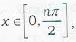n ∈ N, then the greatest value of n is

Solution:
QUESTION: 9

The number of value of x ∈ [0, 2π] that satisfy cot x - cosec x = 2 sin x is

Solution:
QUESTION: 10

If sin a, 1 and cos 2a are in GP then a is equl to

Solution:
QUESTION: 11

If 1/6 sinθ, cosθ and tanθ are in GP then the general value of θ is

Solution:
QUESTION: 12

The general values of x for which cos 2x, 1/2 and sin 2x are in AP are given by

Solution:
QUESTION: 13

The most general values of θ satisfying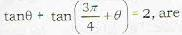Solution:
QUESTION: 14

The most general solutions of the equation sec x - 1 - (√2 - 1) tan x are given by

Solution:
QUESTION: 15

The most general solutions of the equation sec2x √2(1 - tan2x) are given by

Solution:
QUESTION: 16

The most general values of x for which sin x + cos x,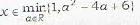are given by

Solution:
QUESTION: 17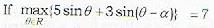then the set of possible values of α is

Solution:
QUESTION: 18

Let α, β, be any two positive values of x for which 2cos x, |cosx| and 1- 3cos2 x are in GP. The minimum value of |α-β| is

Solution:
QUESTION: 19

The sum of all the solutions of the equation cos x.cos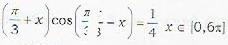is

Solution:
QUESTION: 20

The values of x ∈ [-2π, 2π] such that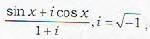is purely imaginary, are given by

Solution: